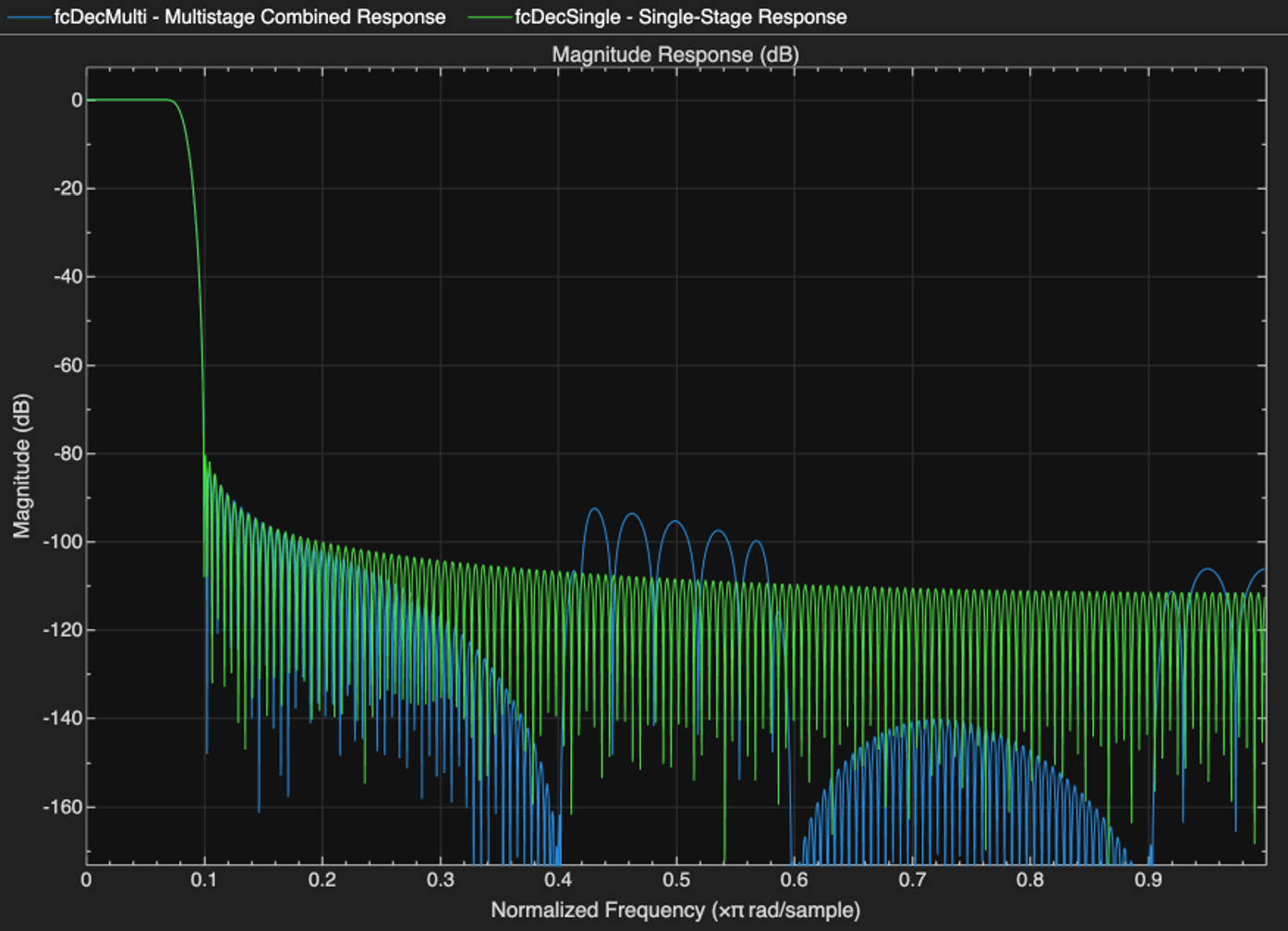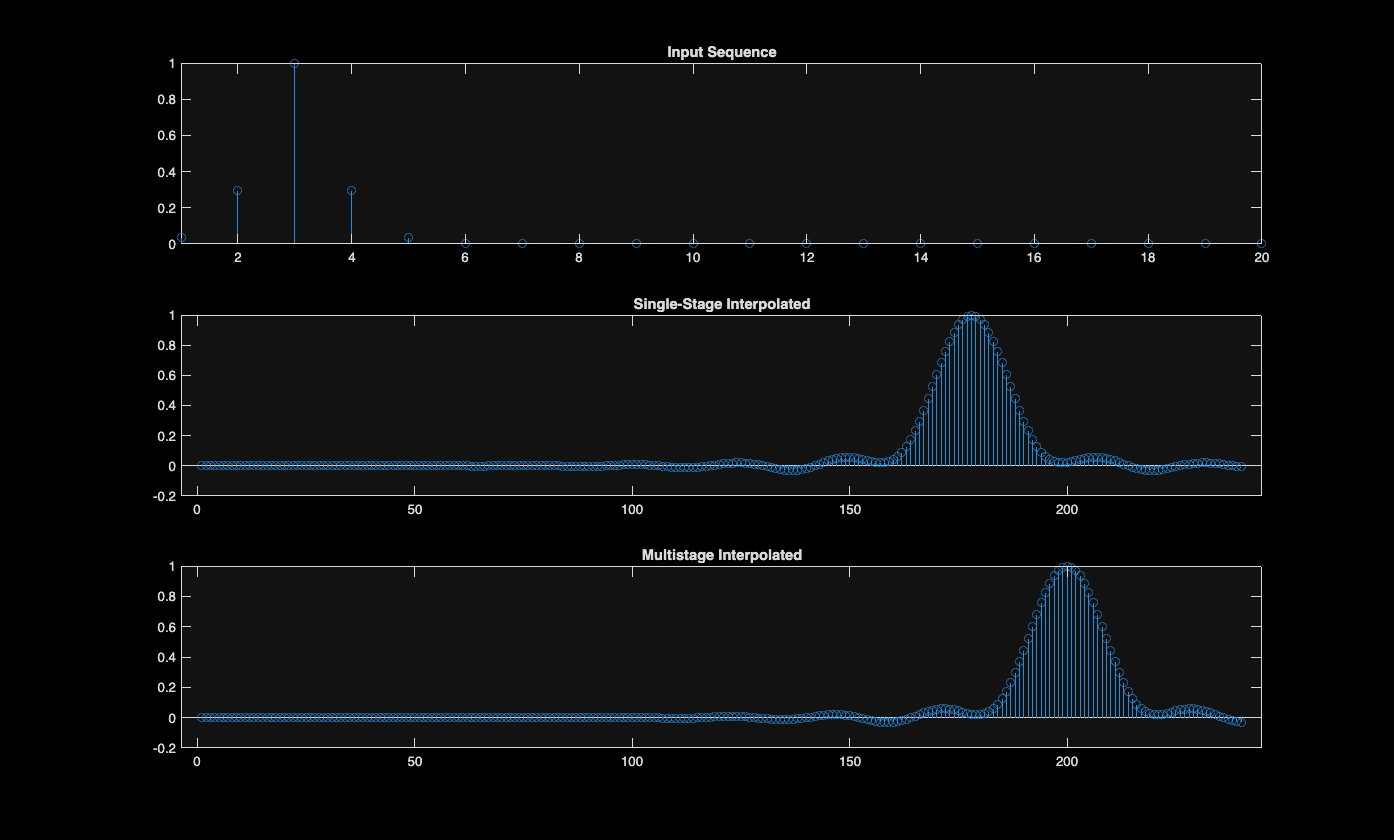# Multistage Rate Conversion

Multistage rate conversion is an approach that splits rate conversion into several stages. For example, instead of decimation by a factor of 18, decimate by factor of 3, followed by another decimation by 3, and and then by a factor of 2. Using multiple stages reduces the computational complexity of filtered rate conversion. Furthermore, if one already has the converter units for the different prime factors, they can be used as building blocks for higher rates. This example will demonstrate multistage rate conversion designs.

### Single-Stage v.s. Multistage Conversion: Cost Analysis

Consider decimation system of rate M=8. One can implement such a system in two ways:

• A single decimator of rate M=8.

• A cascade of three half-rate decimators (M=2)

While the two alternatives effectively have the same decimation factor, they differ in their numerical complexites. Evaluate the cost of implementing a multistage decimator using the cost function, and compare it to the cost of implementing a single-stage decimator.

bDecim2 = designMultirateFIR(1,2); firDecim2_1 = dsp.FIRDecimator(2,bDecim2); firDecim2_2 = dsp.FIRDecimator(2,bDecim2); firDecim2_3 = dsp.FIRDecimator(2,bDecim2); firDecim2cascade = dsp.FilterCascade(firDecim2_1,firDecim2_2,firDecim2_3); cost2cascade = cost(firDecim2cascade) bDecim8 = designMultirateFIR(1,8); firDecim8 = dsp.FIRDecimator(8,bDecim8); cost8 = cost(firDecim8) 
cost2cascade = struct with fields: NumCoefficients: 75 NumStates: 138 MultiplicationsPerInputSample: 21.8750 AdditionsPerInputSample: 21 cost8 = struct with fields: NumCoefficients: 169 NumStates: 184 MultiplicationsPerInputSample: 21.1250 AdditionsPerInputSample: 21 

Cascading three decimators of rate M=2 consumes less memory (states and coefficients) compared to a single-stage decimator of M=8, making the multistage converter more memory efficient. The arithmetic load (operations per sample) of the single-stage and multistage implementation are equivalent. Note that the number of samples drops by half after each decimation stage. In conclusion, it is often better to split the decimation into multiple stages (given that the rate change factor is not a prime number, of course).

There is usually more than one way to factor a (non-prime) conversion rate, and even more degrees of freedom multistage design. The DSP System Toolbox (TM) offers several tools to simplify the design process. We will examine two of them in what follows.

### Using designMultistageDecimator and designMultistageInterpolator functions

The designMultistageInterpolator and designMultistageDecimator functions automatically determine an optimal configuation, that includes determining the number of stages along with their arrangements, lowpass parameters, etc. The result is a filter cascade system object, which encapsualtes all the stages. To illustrate, let us design a decimator of rate M=12.

M = 12; fcDecMulti = designMultistageDecimator(M); info(fcDecMulti) 
ans = 'Discrete-Time Filter Cascade ---------------------------- Number of stages: 3 Stage1: dsp.FIRDecimator ------- Discrete-Time FIR Multirate Filter (real) ----------------------------------------- Filter Structure : Direct-Form FIR Polyphase Decimator Decimation Factor : 2 Polyphase Length : 6 Filter Length : 11 Stable : Yes Linear Phase : Yes (Type 1) Arithmetic : double Stage2: dsp.FIRDecimator ------- Discrete-Time FIR Multirate Filter (real) ----------------------------------------- Filter Structure : Direct-Form FIR Polyphase Decimator Decimation Factor : 2 Polyphase Length : 8 Filter Length : 15 Stable : Yes Linear Phase : Yes (Type 1) Arithmetic : double Stage3: dsp.FIRDecimator ------- Discrete-Time FIR Multirate Filter (real) ----------------------------------------- Filter Structure : Direct-Form FIR Polyphase Decimator Decimation Factor : 3 Polyphase Length : 27 Filter Length : 79 Stable : Yes Linear Phase : Yes (Type 1) Arithmetic : double ' 

This particular design has 3 stages (), where the lowpass of the last stage is the longest.

Repeat the design with a single-stage.

fcDecSingle = designMultistageDecimator(M,'NumStages',1); info(fcDecSingle) 
ans = 'Discrete-Time Filter Cascade ---------------------------- Number of stages: 1 Stage1: dsp.FIRDecimator ------- Discrete-Time FIR Multirate Filter (real) ----------------------------------------- Filter Structure : Direct-Form FIR Polyphase Decimator Decimation Factor : 12 Polyphase Length : 26 Filter Length : 307 Stable : Yes Linear Phase : Yes (Type 1) Arithmetic : double ' 

Compare the cost of the two implementations. Obivously, the multistage approach is more efficient.

costMulti = cost(fcDecMulti) costSingle = cost(fcDecSingle) 
costMulti = struct with fields: NumCoefficients: 69 NumStates: 102 MultiplicationsPerInputSample: 10.1667 AdditionsPerInputSample: 9.3333 costSingle = struct with fields: NumCoefficients: 283 NumStates: 300 MultiplicationsPerInputSample: 23.5833 AdditionsPerInputSample: 23.5000 

Now, let us compare the combined frequency response of the decimation filters. While the filters of the two implementations differ in the stopband, the passband and transition band are nearly identical.

hfv = fvtool(fcDecMulti, fcDecSingle); legend(hfv,'Multistage Combined Response', 'Single-Stage Response');The same methodology applies for designMultistageInterpolator. Create two interpolators (single-stage and multistage) and compare their outputs. Note that the outputs are nearly identical, except a slightly longer latency of the multistage interpolator.

n = (1:20)'; x = (abs(n-5)<=5).*(5-abs(n-5)); L = 12; fcIntrMulti = designMultistageInterpolator(L); fcIntrSingle = designMultistageInterpolator(L,'NumStages',1); xInterpSingle = fcIntrSingle(x); xInterpMulti = fcIntrMulti(x); release(fcIntrMulti); release(fcIntrSingle); subplot(3,1,1); stem(x); xlim([1,20]); title('Input Sequence'); subplot(3,1,2); stem(xInterpSingle); title('Single-Stage Interpolated') subplot(3,1,3); stem(xInterpMulti); title('Multistage Interpolated')### The dsp.SampleRateConverter System Object

The dsp.SampleRateConverter system object provides a convenient interface for arbitrary rate conversion, combining interpolation and decimation as needed.

src = dsp.SampleRateConverter('InputSampleRate',18,'OutputSampleRate',16,'Bandwidth',13); info(src) 
ans = 'Overall Interpolation Factor : 8 Overall Decimation Factor : 9 Number of Filters : 1 Multiplications per Input Sample: 24.333333 Number of Coefficients : 219 Filters: Filter 1: dsp.FIRRateConverter - Interpolation Factor: 8 - Decimation Factor : 9 ' 

The different stages can be extracted with the getFilters function:

firs = getFilters(src) 
firs = dsp.FilterCascade with properties: Stage1: [1x1 dsp.FIRRateConverter] 

We can also specify absolute frequencies (rather than ratios). For example, the dsp.SampleRateConverter object can convert audio data sample rate from 48 kHz to 44.1 kHz.

src = dsp.SampleRateConverter('InputSampleRate',48000,'OutputSampleRate',44100); [L,M] = getRateChangeFactors(src); firs = getFilters(src); reader = dsp.AudioFileReader('audio48kHz.wav','SamplesPerFrame',4*M); x = reader(); xr = src(x); % Obtain the rate conversion FIR b = firs.Stage1.Numerator; % Calculate the resampling delay i0 = floor(length(b)/2)/L; figure; hold on; stem((1:length(x))+i0,x); stem(linspace(1,length(x),length(xr)),xr,'r'); hold off; legend('Input Audio','Resampled Audio'); xlim([150,200]) release(reader);### Simplification by Rate Conversion Slack

Conversion ratios like(used in the previous section) requires a large upsampling and downsampling ratios, as even its reduced form is. The filters required for such a conversion are fairly long, introducing a significant latency in addition to the memory and computational load.

cost(src) 
ans = struct with fields: NumCoefficients: 8587 NumStates: 58 MultiplicationsPerInputSample: 53.6688 AdditionsPerInputSample: 52.7500 

We can mitigate the costly conversion by approximating the rate conversion factor. For example,The deviation of 100Hz is small, only 0.23 % of the absolute frequencies. The dsp.SampleRateConverter can automatically approximate the rate conversion factor by allowing the output frequency to be perturbed. The perturbation tolerance is specified through the 'OutputRateTolerance' property. The default tolerance is 0, meaning, no slack. In other words, slack means the deviation from the specified output rate value. Clearly, the approximated rate conversion has much smaller computational cost, and suffices for many applications, such as standard definition audio processing.

src_approx = dsp.SampleRateConverter('InputSampleRate',48000,... 'OutputSampleRate',44100,'Bandwidth',13,... 'OutputRateTolerance',0.01); [L_approx,M_approx] = getRateChangeFactors(src_approx) cost(src_approx) 
L_approx = 11 M_approx = 12 ans = struct with fields: NumCoefficients: 61 NumStates: 5 MultiplicationsPerInputSample: 5.0833 AdditionsPerInputSample: 4.1667 

## SupportGet trial now